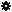﻿ Object Model: EdgeCollection.NeighborVertices

EdgeCollection.NeighborVertices

## EdgeCollection.NeighborVerticesv3.0

### Description

Returns a collection of Vertex object for all vertex neighbors within a given distance.

### C# Syntax

 ```VertexCollection EdgeCollection.NeighborVertices( Int32 in_lDistance ); ```

### Scripting Syntax

 ```oReturn = EdgeCollection.NeighborVertices( [Distance] ); ```

### Parameters

Parameter Type Description
Distance Integer Distance is an integer value representing the degree of neighborhood (eg: degree=2 for an edge means its adjacent edges plus the adjacent edges of the adjacent edges).

Default Value: 1

### Examples

#### VBScript Example

 ```set oCube = ActiveSceneRoot.AddGeometry( "Cube", "MeshSurface" ) set oGeometry = oCube.ActivePrimitive.Geometry set oVertices = oGeometry.Vertices set oNeighborEdges = oVertices(0).NeighborEdges(1) set oNeighbors = oNeighborEdges.NeighborVertices( 1 ) str = "Vertices neighbors of this EdgeCollection are :" for each n in oNeighbors str = str & " " & n.index next logmessage str ```